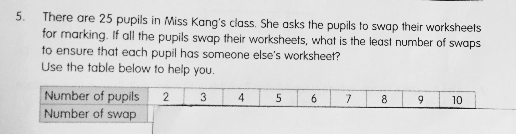QuestionAnyone help me with attached question

Thanks

 No. of pupils 2 3 4 5 6 7 8 9 10 No. of swap 1 2 2 3 3 4 4 5 5

Consider the instance of 7 pupils, the swaps will be

A ↔ B

C ↔ D

E ↔ F

Student G will just swap with any of A, B, C, D, E, F. Therefore, 4 swaps.

If number of pupils is even, then number of swaps = (no. of students) ÷ 2

If number of pupils is odd, then number of swaps = (no. of students + 1) ÷ 2

For 25 pupils, number of swaps = (25 + 1) ÷ 2 = 13

0 Replies 1 Like ✔Accepted Answer

number of pupils : number of swaps
2 : 1
3 : 2
4 : 2
5 : 3
6 : 3

25 : 13

Ans : 13 swaps.

0 Replies 1 Like

When answering questions of this type, the parent must be very clear of the skillset that the examiner is testing. So for this, clearly it will have to be using the child’s inferential skills for pattern recognition

The best way to tackle such problems, will be getting the student to make the linkage to patterns. Once the child understands that it is a pattern question, it will immediately trigger their solving mechanism for such questions.

0 Replies 1 Like

Is there any number given in the number of swap row?

My interpretation of this question is,

when there are 2 students, 1 swap is needed.

when there are 3 students, 2 swaps are needed. (A-B and then A-C)

when there are 4 students, 2 swaps are needed too. (A-B and C-D)

when there are 5 students, 3 swaps are needed. (A-B, C-D and one of them swap with E)

In conclusion…

for even number, the number of swaps needed is N÷2

and for odd number, the number of swaps needed is (N+1)÷2

So, for 25 students , swap needed is (25+1)÷2=13

0 Replies 1 Like